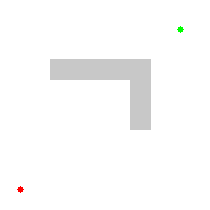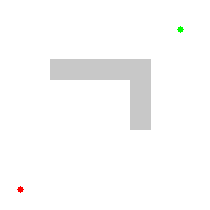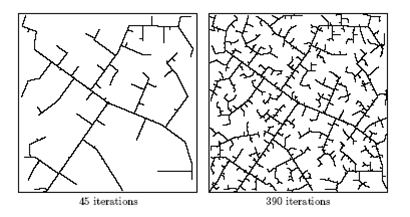### Thanks for stopping by! Please note that this page will not be updated anymore and all content has been moved into an open-source textbook. The books is available open-source on github, compiled as PDF, and in print on Amazon.com.

Path-planning is an important primitive for autonomous mobile robots that lets robots find the shortest – or otherwise optimal – path between two points. Otherwise optimal paths could be paths that minimize the amount of turning, the amount of braking or whatever a specific application requires. Algorithms to find a shortest path are important not only in robotics, but also in network routing, video games and gene sequencing.

Path-planning requires a map of the environment and the robot to be aware of its location with respect to the map. We will assume for now that the robot is able to localize itself,  is equipped with a map, and capable of avoiding temporary obstacles on its way. How to create a map, how to localize a robot, and how to deal with uncertain position information will be major foci of the reminder of this  class. The goals of this lecture are

• introduce suitable map representations
• explain basic path-planning algorithms  ranging from Dijkstra, to A*, D* and RRT
• introduce variations of the path-planning problem, such as coverage path planning
Map representations
In order to plan a path, we somehow need to represent the environment in the computer. We differentiate between two complementary approaches: discrete and continuous approximations. In a discrete approximation, a map is sub-divided into chunks of equal (e.g., a grid or hexagonal map) or differing sizes (e.g., rooms in a building). The latter maps are also known as topological maps. Discrete maps lend themselves well to a graph representation. Here, every chunk of the map corresponds to a vertex (also known as “node”), which are connected by edges, if a robot can navigate from one vertex to the other. For example a road-map is a topological map, with intersections as vertices and roads as edges. Computationally, a graph might be stored as an adjacency or incidence list/matrix. A continuous approximation requires the definition of inner (obstacles) and outer boundaries, typically in the form of a polygon, whereas paths can be encoded as sequences of real numbers. Discrete maps are the dominant representation in robotics.
Currently the most common map is the occupancy grid map. In a grid map, the environment is discretized into squares of arbitrary resolution, e.g. 1cm x 1cm, on which obstacles are marked. In a probabilistic occupancy grid, grid cells can also be marked with the probability that they contain an obstacle. This is particularly important when the position of the robot that senses an obstacle is uncertain. Disadvantages of grid maps are their large memory requirements as well as computational time to traverse data structures with large numbers of vertices. A solution to the latter problem are topological maps that encode entire rooms as vertices and use edges to indicate navigable connections between them. There is no silver bullet, and each application might require a different solution that could be a combination of different map types.
There exist also every possible combination of discrete and continuous representation. For example, roadmaps for GPS systems are stored as topological maps that store the GPS coordinates of every vertex.
Path-Planning Algorithms
The problem to find a “shortest” path from one vertex to another through a connected graph is of interest in multiple domains, most prominently in the internet, where it is used to find an optimal route for a data packet. The term “shortest” refers here to the minimum cumulative edge cost, which could be physical distance (in a robotic application), delay (in a networking application) or any other metric that is important for a specific application.Animation of Dijkstra’s algorithm for robotic path planning, (c) Subhrajit Bhattachary

One of the earliest and simplest algorithms is Dijkstra’s algorithm. Starting from the initial vertex where the path should start, the algorithm marks all direct neighbors of the initial vertex with the cost to get there. It then proceeds from the vertex with the lowest cost to all of its adjacent vertices and marks them with the cost to get to them via itself if this cost is lower. Once all neighbors of a vertex have been checked, the algorithm proceeds to the vertex with the next lowest cost. A more detailed description of this algorithm is provided here. Once the algorithm reaches the goal vertex, it terminates and the robot can follow the edges pointing towards the lowest edge cost.

Looking at the animation to the right shows that the algorithm performs a lot of computations that are “obviously” not going in the right direction. They are obvious because we have a birds-eye view of the environment, seeing the location of the obstacle, and we know the approximate direction of the goal. The latter extra knowledge that we have can be encoded using a heuristic function, a fancier word for a “rule of thumb”. For example, we could give priority to nodes that have a lower estimated distance to the goal than others. For this, we would mark every node not only with the actual distance that it took us to get there (as in Dijkstra’s algorithm), but also with the estimated cost “as the crows flies”, for example by calculating the Euclidean distance or the Manhattan distance between the vertex we are looking at and the goal vertex. This algorithm is known as A*. Depending on the environment, A* might accomplish search much faster than Dijkstra’s algorithm. The algorithm is provided in pseudo-code here.Illustration of A* planning, (c) Subhrajit Bhattachary

An extension of A* that addresses the problem of expensive re-planning when obstacles appear in the path of the robot, is known as D*. Unlike A*, D* starts from the goal vertex and has the ability to change the costs of parts of the path that include an obstacle. This allows D* to re-plan around an obstacle while maintaining most of the already calculated path.

A* and D* become computationally expensive when either the search space is large, e.g., due to a fine-grain resolution required for the task, or the dimensions of the search problem are high, e.g. when planning for an arm with multiple degrees of freedom. A more recent development known as Rapidly-Exploring Random Trees (RRT) addresses this problem by using a randomized approach that aims at quickly exploring a large area of the search space with iterative refinement. For this, the algorithm selects a random point in the environment and connects it to the initial vertex. Subsequent random points are then connected to the closest vertex in the emerging graph. The graph is then connected to the goal node, whenever a point in the tree comes close enough given some threshold. Although generally a coverage algorithm (see also below), RRT can be used for path-planning by maintaining the cost-to-start on each added point, and biasing the selection of points to occasionally falling close to the goal. RRT can also be used to take into account non-holonomic contraints of a specific platform when generating the next random way-point. One way to implement this is to calculate the next random point by applying random values to the robot’s actuators and use the forward kinematics to calculate the next point. Most recently, variations of RRT have been proposed that will eventually find an optimal solution. Nevertheless, although RRT quickly finds some solution, smooth paths usually require additional search algorithms that start from an initial estimate provided by RRT.Example RRT search. (c) LaValle and Kuffner

Robot embodiment

In order to deal with the physical embodiment of the robot, which complicates the path-planning process, the robot is reduced to a point-mass and all the obstacles in the environment are grown by half of the longest extension of the robot from its center. This representation is known as configuration space as it reduces the representation of the robot to its x and y coordinates in the plane. Most planning algorithms also do not consider the orientation of the robot. If this is desired, an additional dimension needs to be introduced into the configuration space.

In order to see how finding the shortest path can be applied to a 2-link manipulator, and how obstacles in x-y space get projected into the configuration space of such a robot, check out Ken Goldberg’s configuration space Java applet.

Other path-planning applications
Once the environment has been discretized into a graph (note, a graph is only one possible representation), we can employ other algorithms from graph theory to plan desirable robot trajectories. For example, floor coverage can be achieved by performing a depth-first search (DFS) or a breadth-first-search (BFS) on a graph where each vertex has the size of the coverage tool of the robot. “Coverage” is not only interesting for cleaning a floor: the same algorithms can be used to perform an exhaustive search of a configuration space, such as in the example seen in Lecture #3, where we plotted the error of a manipulator arm in reaching a desired position over its configuration space. Finding a minimum in this plot using an exhaustive search solves the inverse kinematics problem. Similarly, the same algorithm can be used to systematically follow all links on a website till a desired depth (or actually retrieving the entire world-wide web).
Doing a DFS or a BFS might generate efficient coverage paths, but they are far from optimal as many vertices might be visited twice. A path that connects all vertices in a graph but passes every vertex only once is known as a Hamiltonian Path. A Hamiltonian path that returns to its starting vertex is known as a Hamiltonian Cycle. This problem is also known as the Traveling Salesman Problem (TSP), in which a route needs to be calculated that visits every city on his tour only once and is known to be NP Complete.
Exercise: Think about the computational and memory (space) requirements of DFS, BFS, and any exact TSP algorithm of your choice and rank them. What kind of trade-off are you making as a designer when deciding between these algorithms to develop a reliable floor cleaning robot?
Take-home lessons
• The first step in path planning is choosing a map representation that is appropriate to the application
• The second step is to reduce the robot to a point-mass, which allows planning in the configuration space
• This allows the application of generic shortest path finding algorithms, which have applications in a large variety of domains, not limited to robotics.